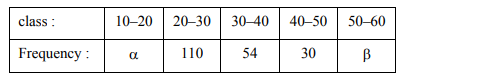# Consider the following frequency distribution :`
Question:

Consider the following frequency distribution :If the sum of all frequencies is 584 and median is 45 , then $|\alpha-\beta|$ is equal to__________.

Solution:

$\because$ Sum of frequencies $=584$

$\Rightarrow \alpha+\beta=390$

Now, Median is at $\frac{584}{2}=292^{\text {th }}$

$\because$ Median $=45($ lies in class $40-50)$

$\Rightarrow \alpha+110+54+15=292$

$\Rightarrow \alpha=113, \beta=277$

$\Rightarrow|\alpha-\beta|=164$